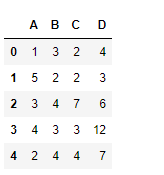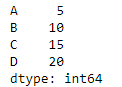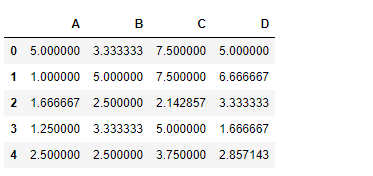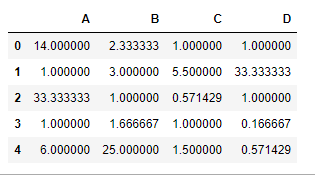GFG App
Open AppBrowser
Continue

# Python | Pandas dataframe.rdiv()

Python is a great language for doing data analysis, primarily because of the fantastic ecosystem of data-centric python packages. Pandas is one of those packages and makes importing and analyzing data much easier.

Pandas` dataframe.rdiv()` function compute Floating division of dataframe and other, element-wise (binary operator rtruediv). Other object could be a scalar, pandas series or pandas dataframe. This function is essentially same as doing ` other / dataframe ` but with support to substitute a fill_value for missing data in one of the inputs.

Syntax: DataFrame.rdiv(other, axis=’columns’, level=None, fill_value=None)

Parameters :
other : Series, DataFrame, or constant
axis : For Series input, axis to match Series index on
level : Broadcast across a level, matching Index values on the passed MultiIndex level
numeric_only : Include only float, int, boolean data. Valid only for DataFrame or Panel objects
fill_value : Fill existing missing (NaN) values, and any new element needed for successful DataFrame alignment, with this value before computation. If data in both corresponding DataFrame locations is missing the result will be missing

Returns : result : DataFrame

Example #1: Use `rdiv()` function to divide a series with a dataframe element-wise

 `# importing pandas as pd ` `import` `pandas as pd ` ` `  `# Creating the dataframe  ` `df ``=` `pd.DataFrame({``"A"``:[``1``, ``5``, ``3``, ``4``, ``2``], ` `                   ``"B"``:[``3``, ``2``, ``4``, ``3``, ``4``],  ` `                   ``"C"``:[``2``, ``2``, ``7``, ``3``, ``4``], ` `                   ``"D"``:[``4``, ``3``, ``6``, ``12``, ``7``]}) ` ` `  `# Print the dataframe ` `df `Lets create a series

 `# importing pandas as pd ` `import` `pandas as pd ` ` `  `# Create a series ` `sr ``=` `pd.Series([``5``, ``10``, ``15``, ``20``], index ``=``[``"A"``, ``"B"``, ``"C"``, ``"D"``]) ` ` `  `# Print the series ` `sr `Let’s use the `dataframe.rdiv()` function to divide the series with a dataframe

 `# perform division of series with  ` `# dataframe element-wise over the column axis ` `df.rdiv(sr, axis ``=` `1``) `

Output :Example #2: Use `rdiv()` function to divide one dataframe with other which contains `NaN` value.

 `# importing pandas as pd ` `import` `pandas as pd ` ` `  `# Creating the first dataframe  ` `df1 ``=` `pd.DataFrame({``"A"``:[``1``, ``5``, ``3``, ``4``, ``2``], ` `                    ``"B"``:[``3``, ``2``, ``4``, ``3``, ``4``],  ` `                    ``"C"``:[``2``, ``2``, ``7``, ``3``, ``4``], ` `                    ``"D"``:[``4``, ``3``, ``6``, ``12``, ``7``]}) ` ` `  `# Creating the second dataframe ` `df2 ``=` `pd.DataFrame({``"A"``:[``14``, ``5``, ``None``, ``4``, ``12``], ` `                    ``"B"``:[``7``, ``6``, ``4``, ``5``, ``None``], ` `                    ``"C"``:[``2``, ``11``, ``4``, ``3``, ``6``],  ` `                    ``"D"``:[``4``, ``None``, ``6``, ``2``, ``4``]}) ` ` `  `# divide df2 by df1 element-wise ` `# Fill all the missing values by 100 ` `df1.rdiv(df2, fill_value ``=` `100``) `

Output :My Personal Notes arrow_drop_up